Check Important Questions and Chapter Summary -https://you.tube/Real-Numbers-Class-10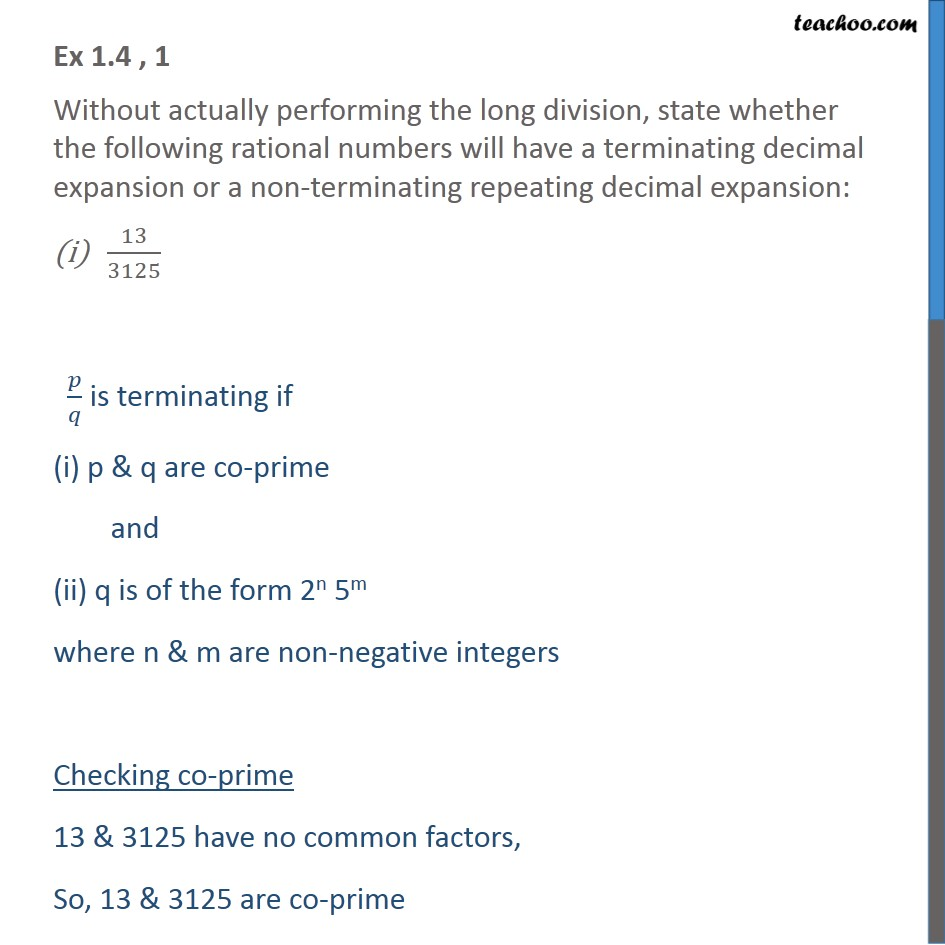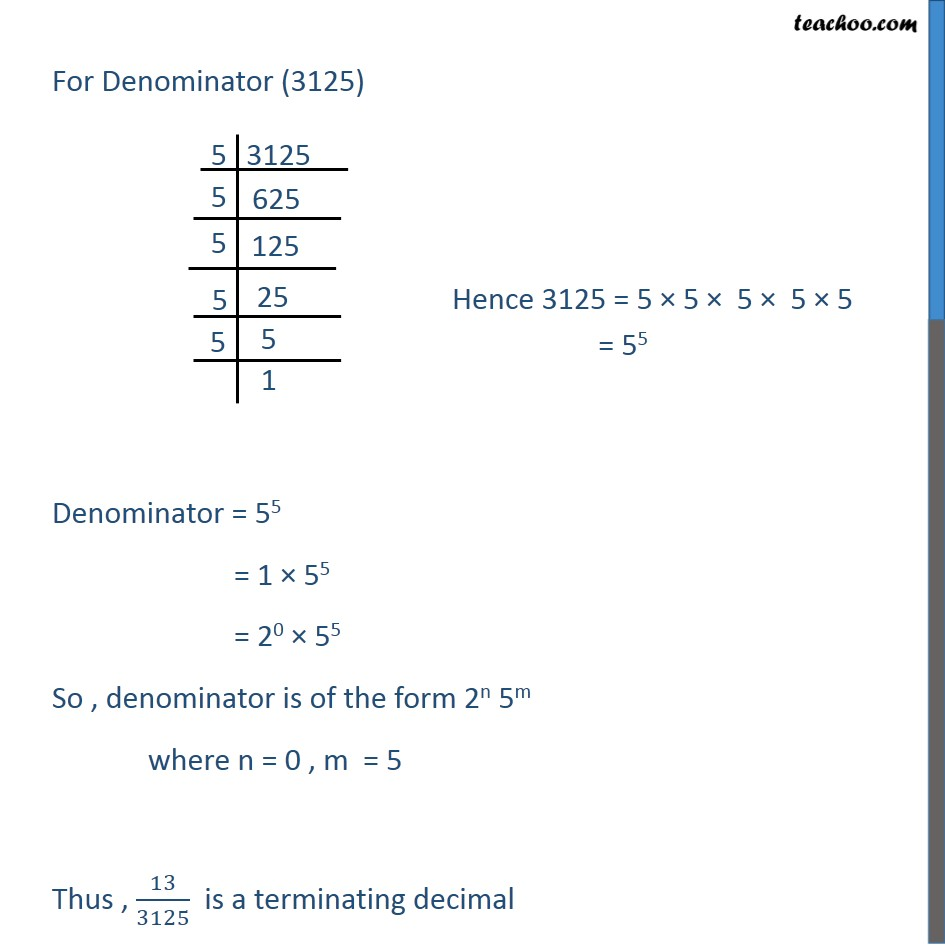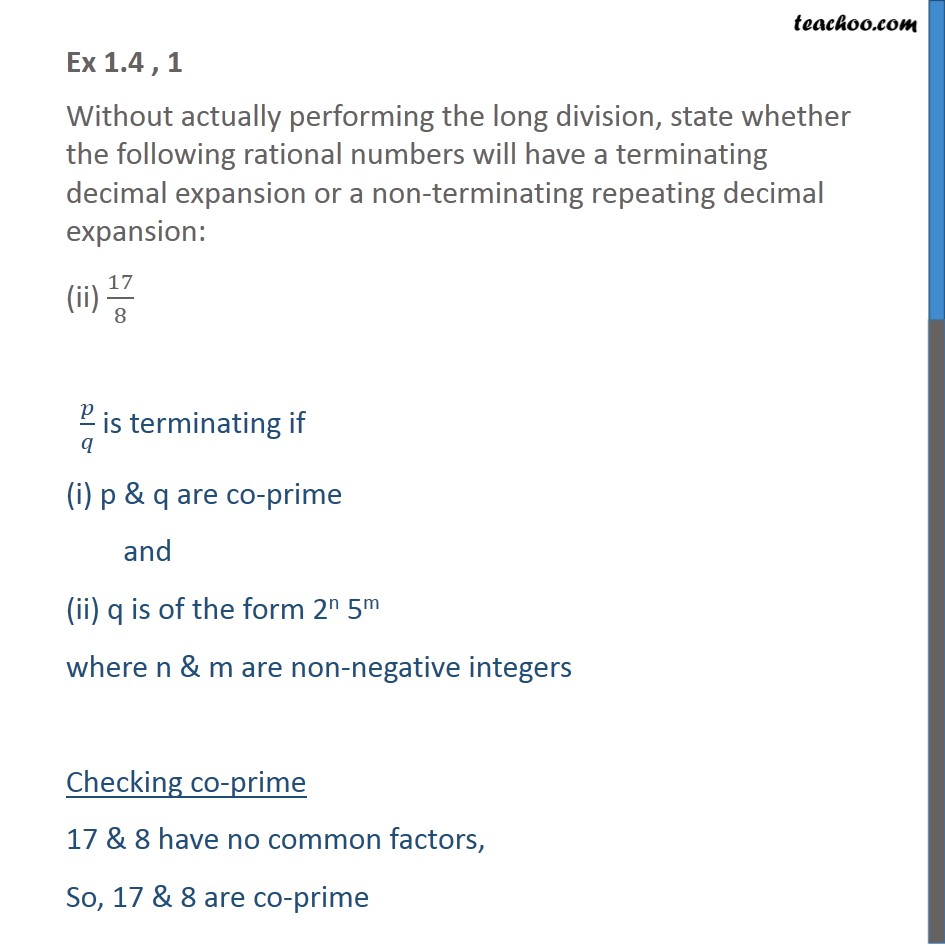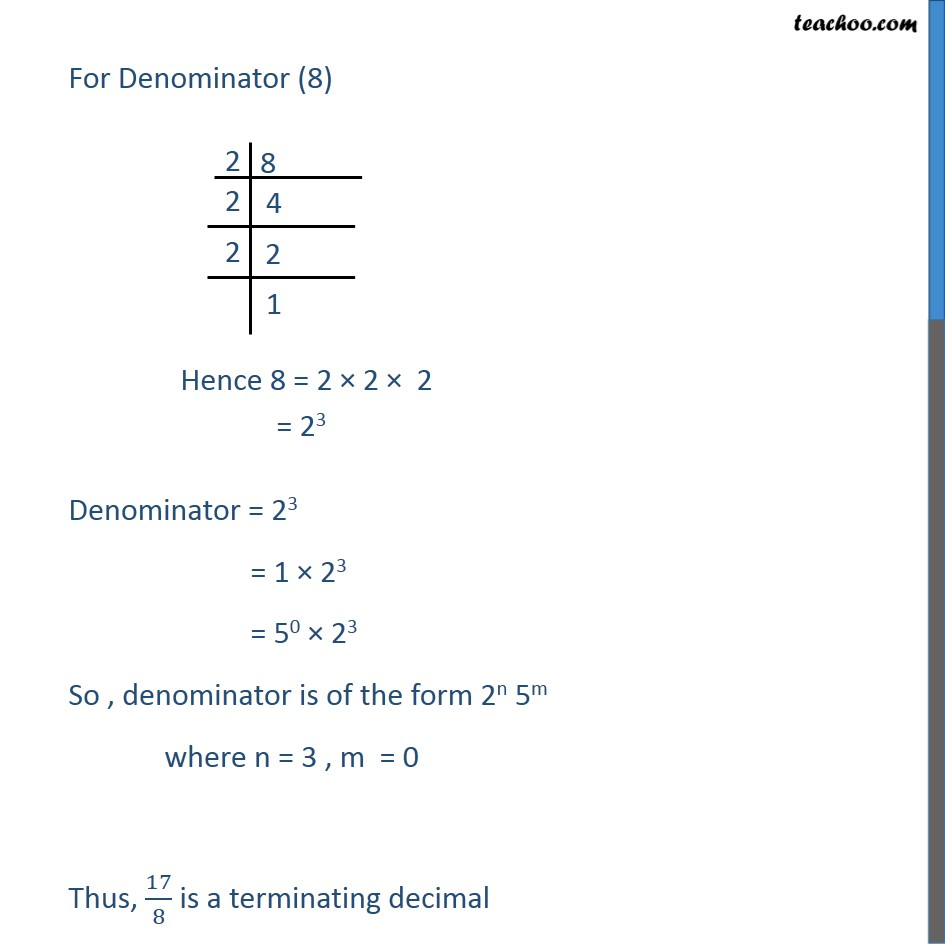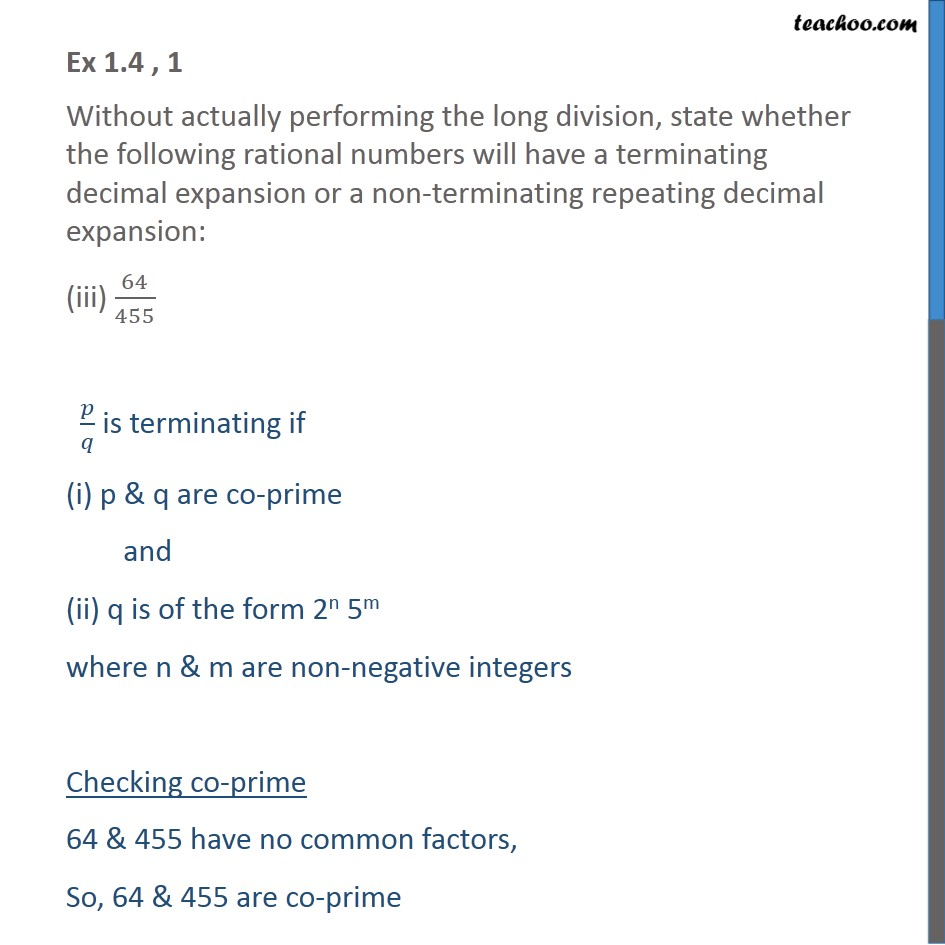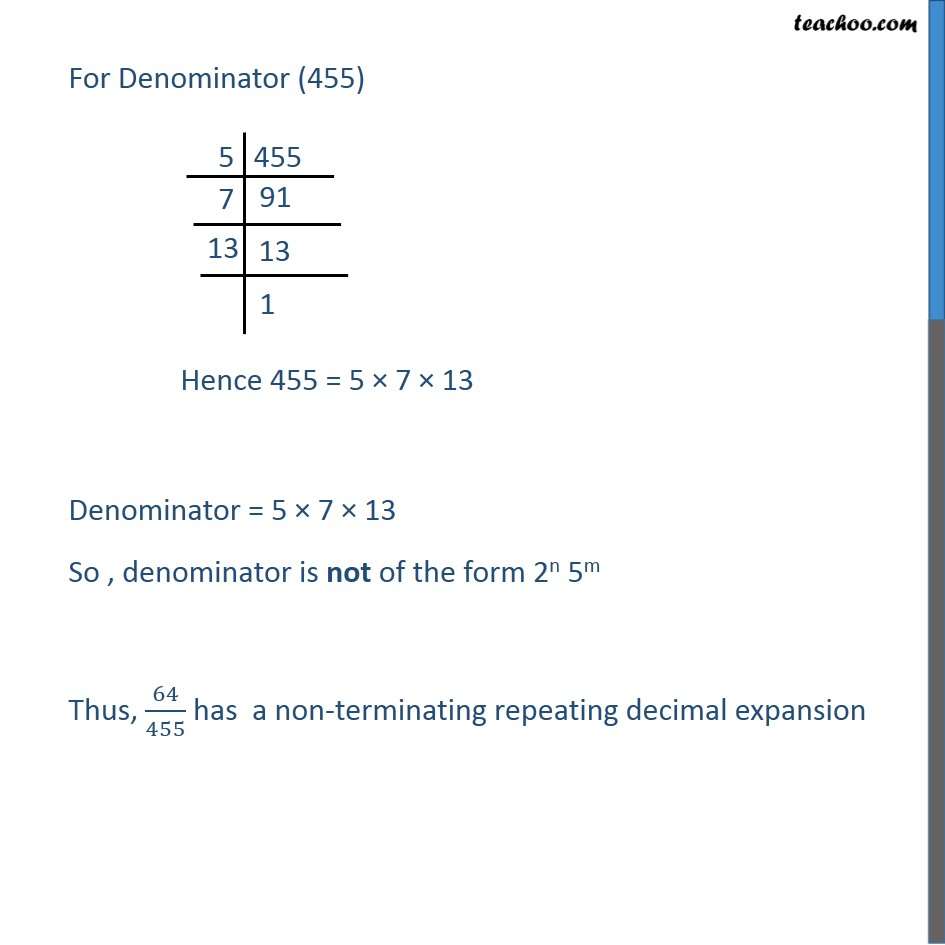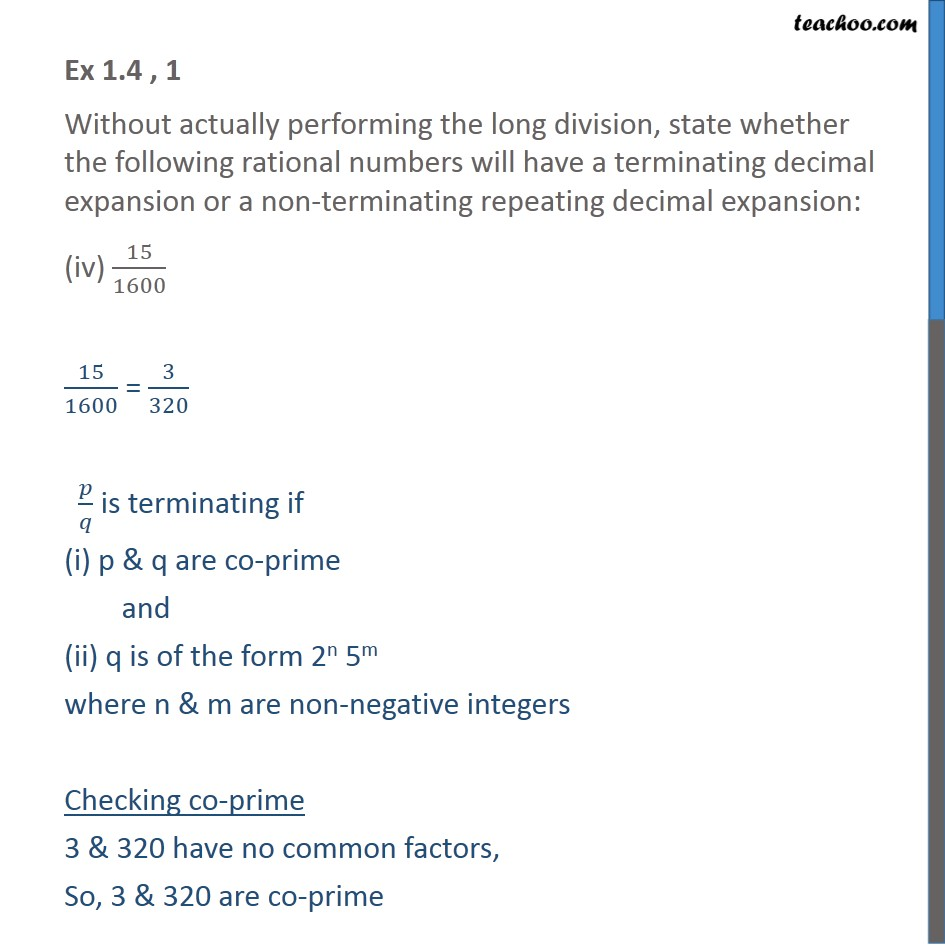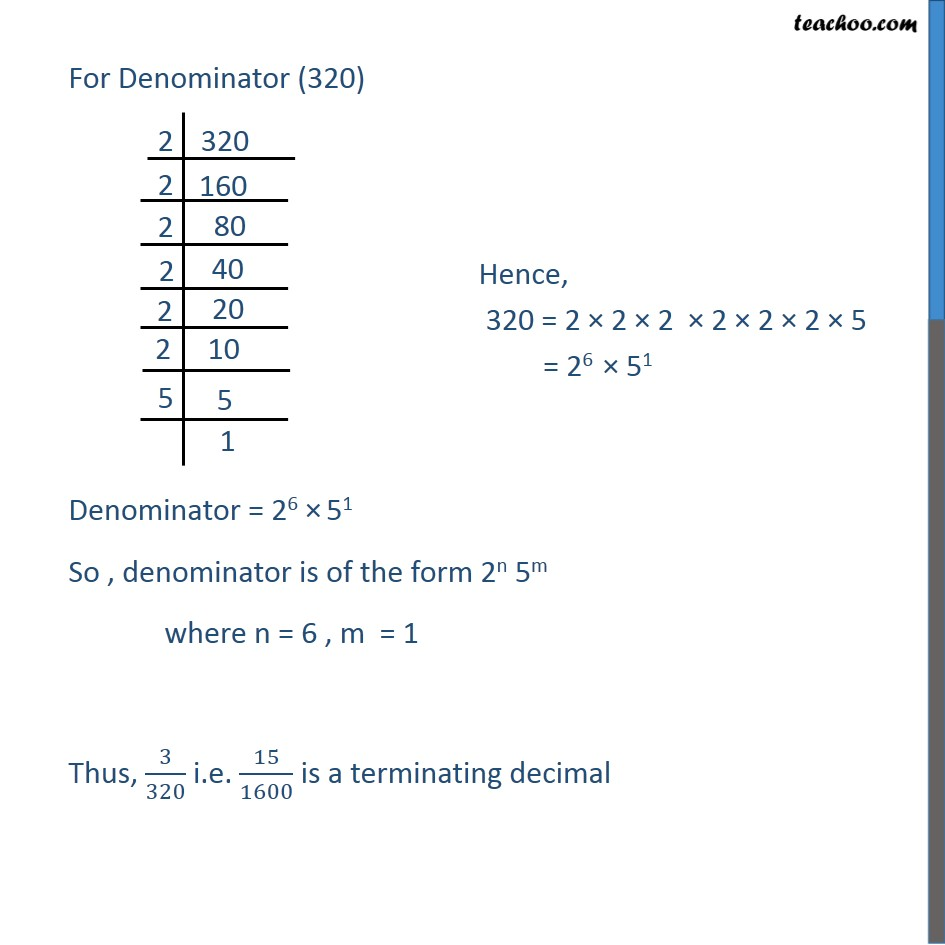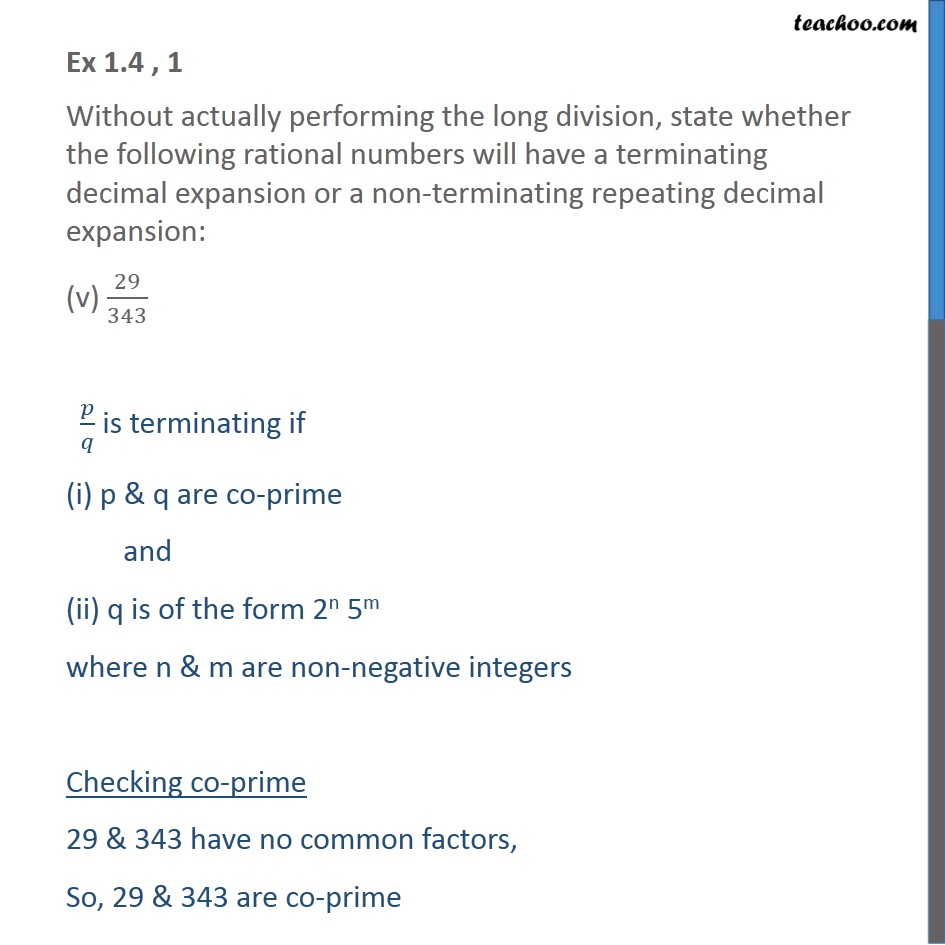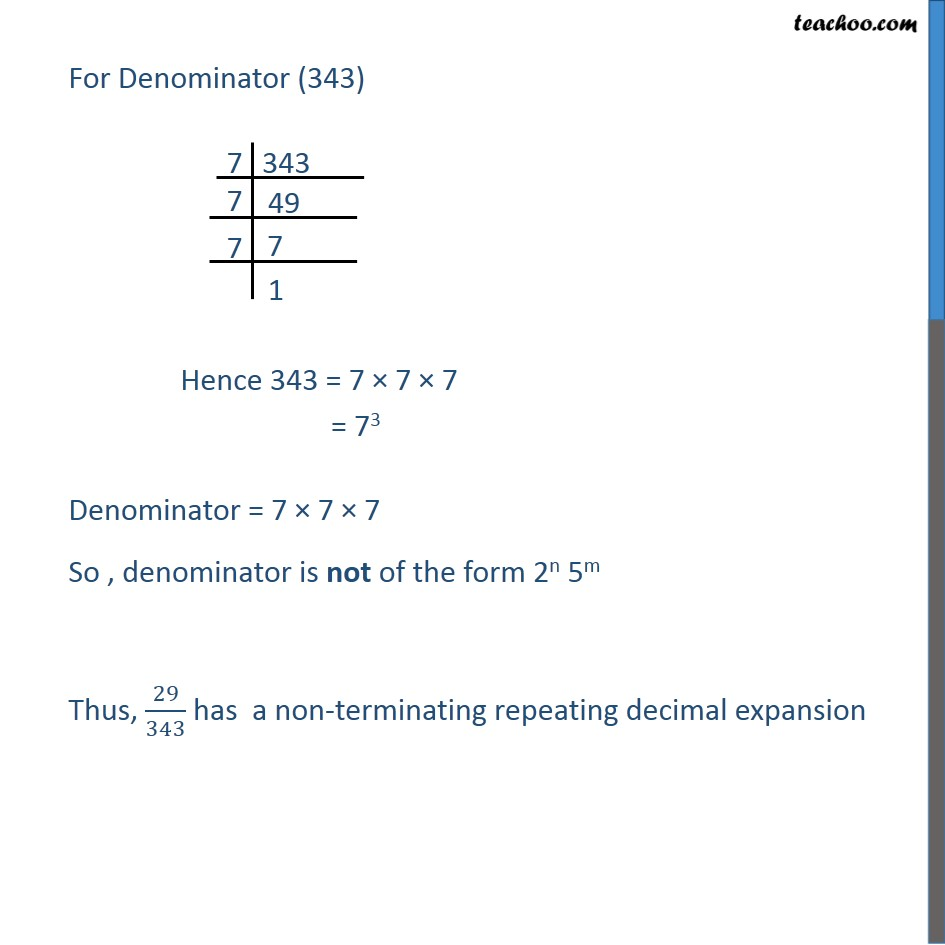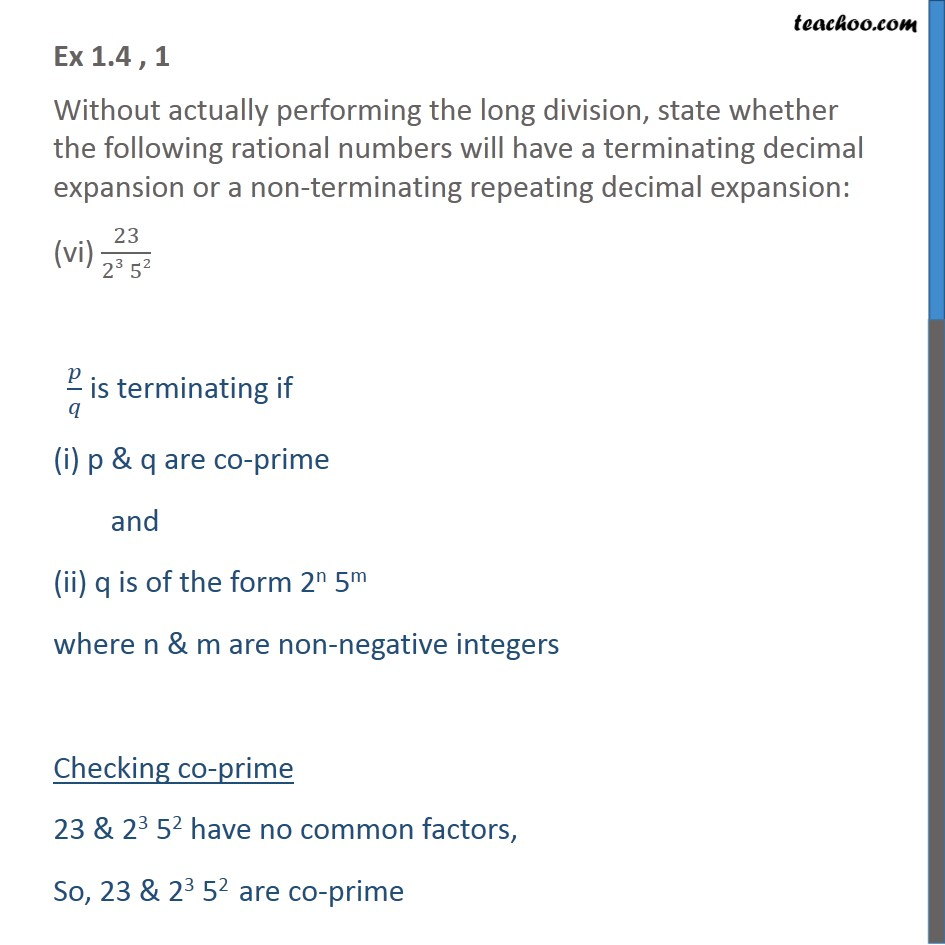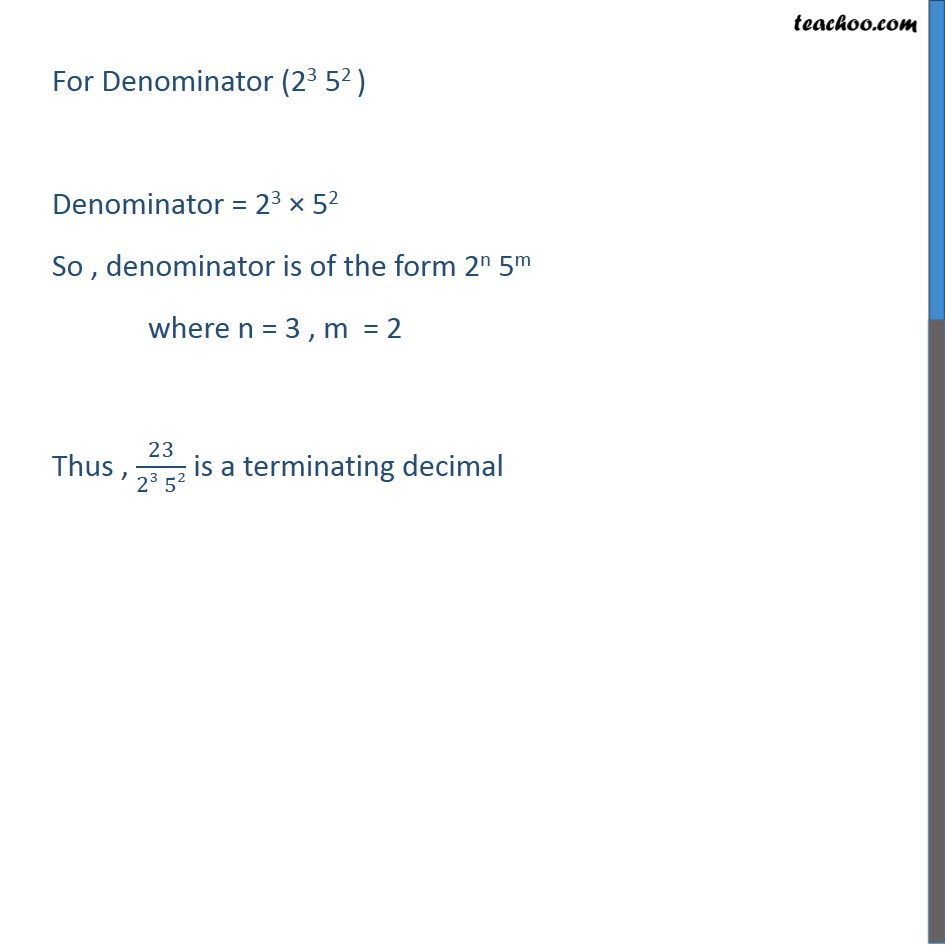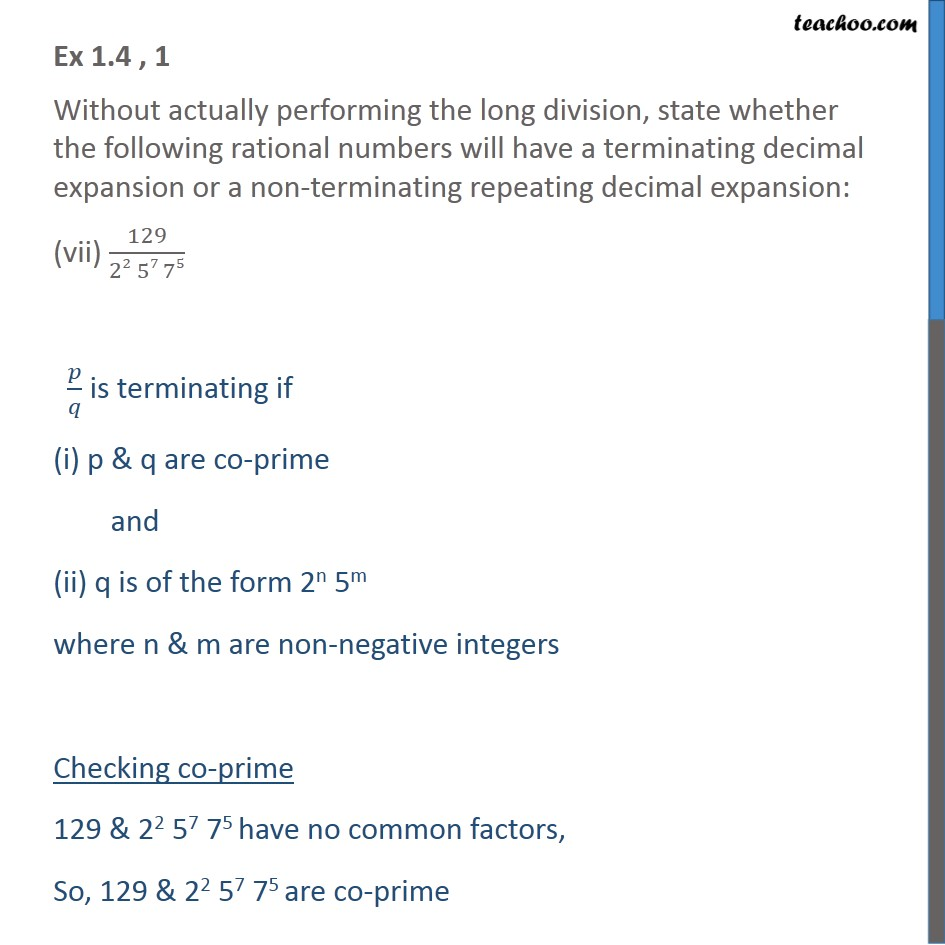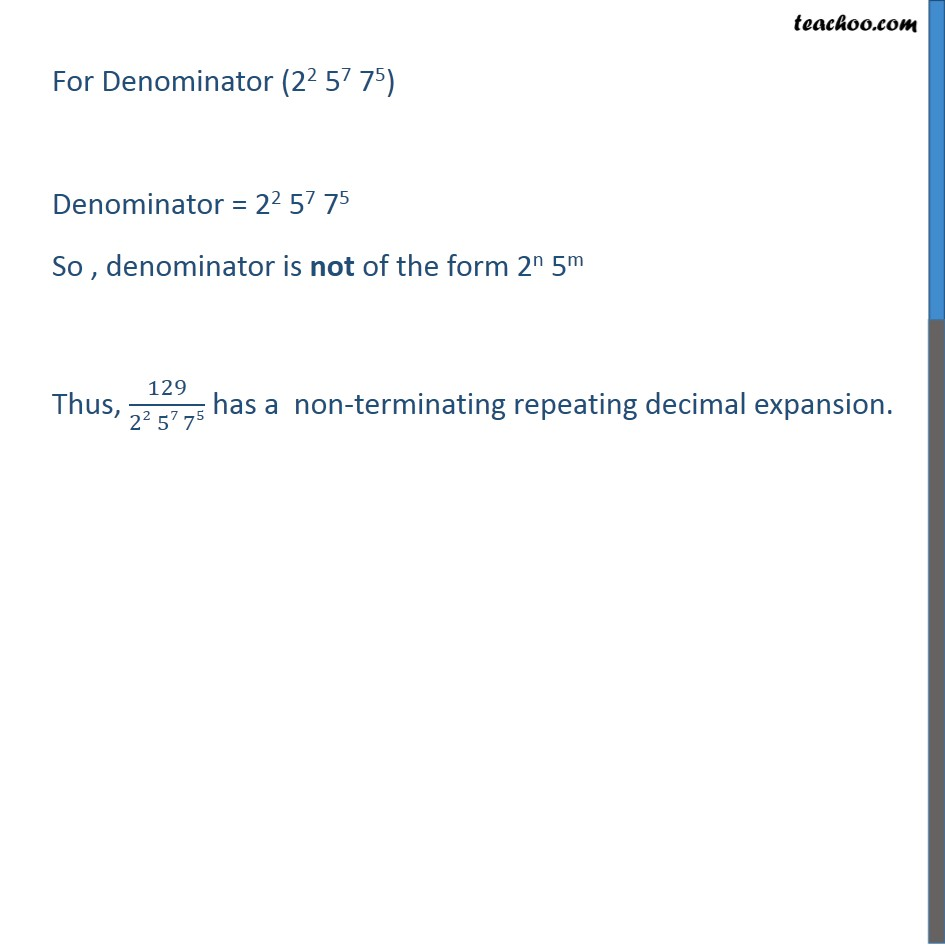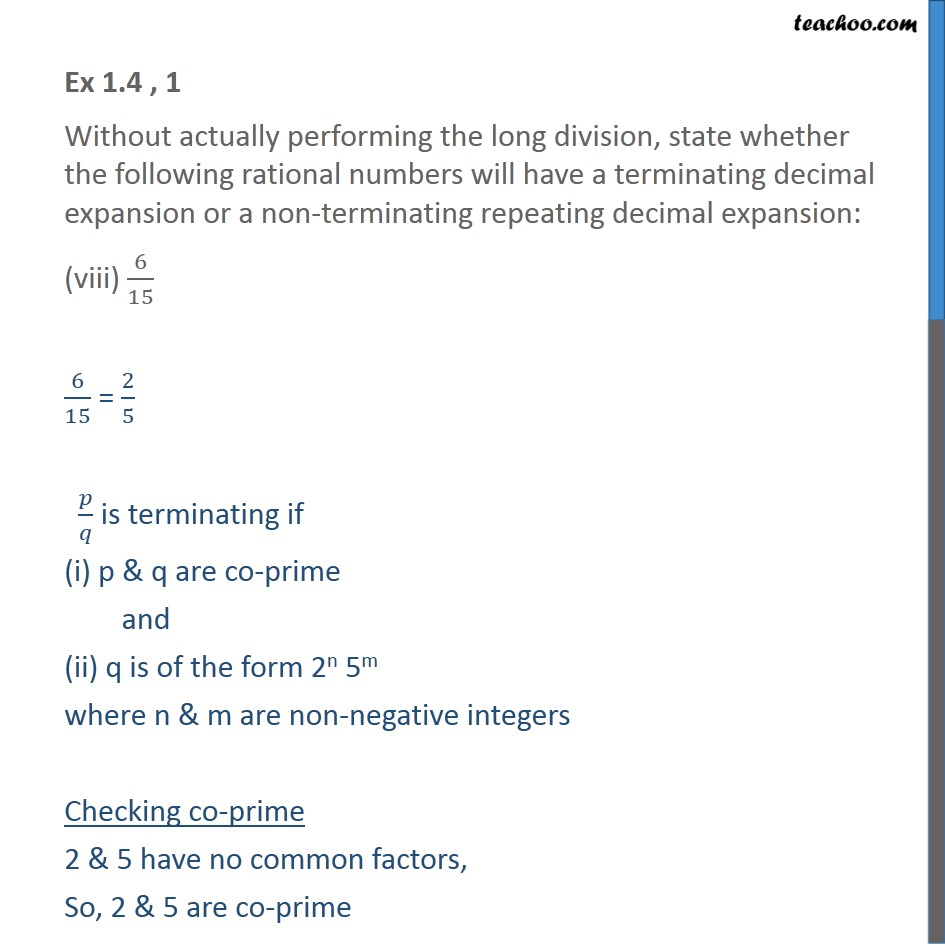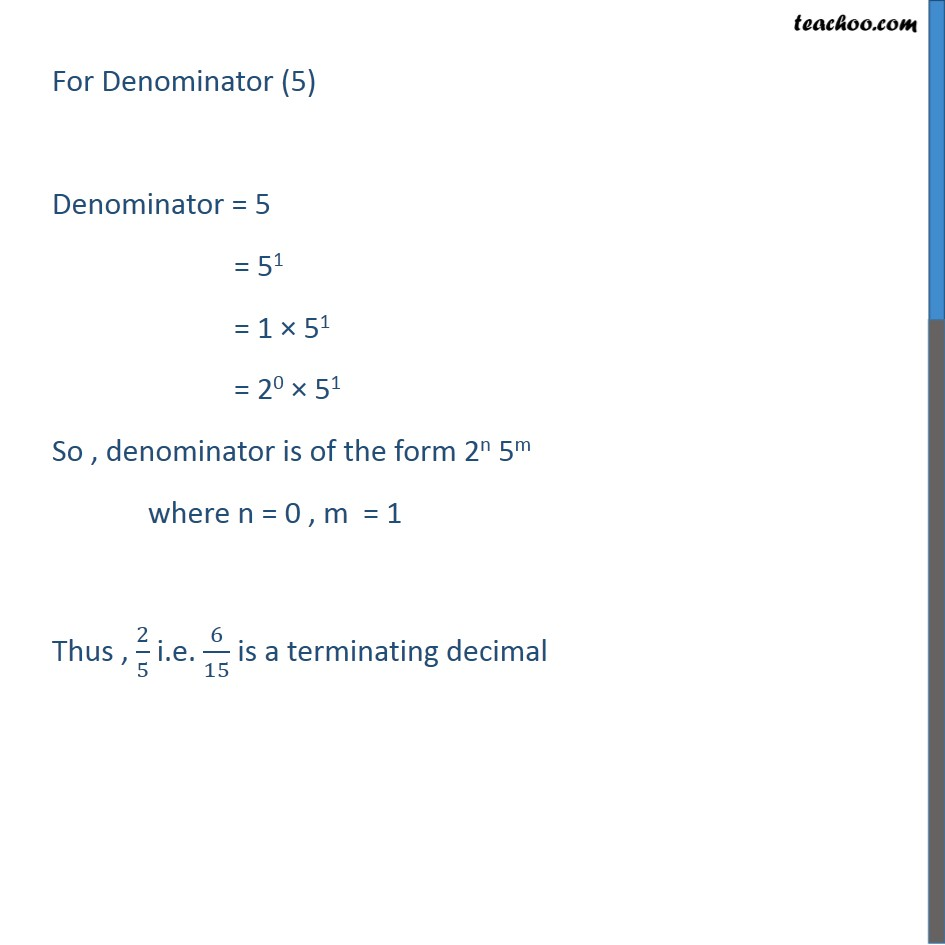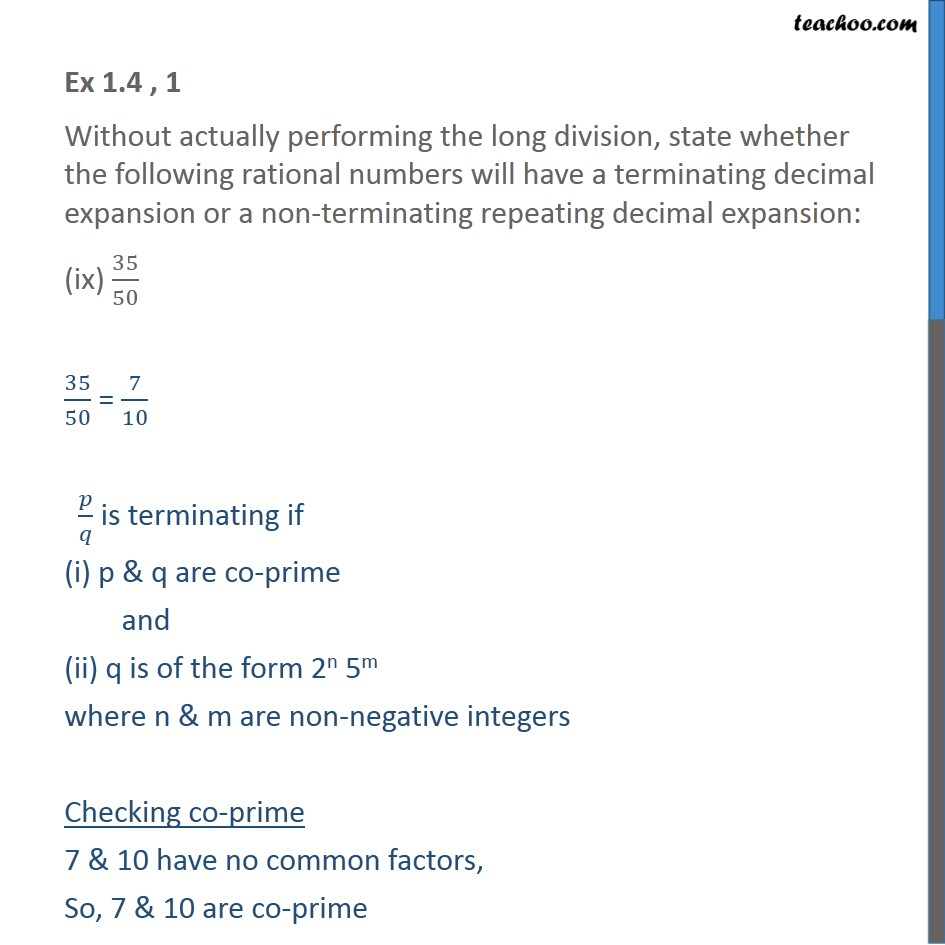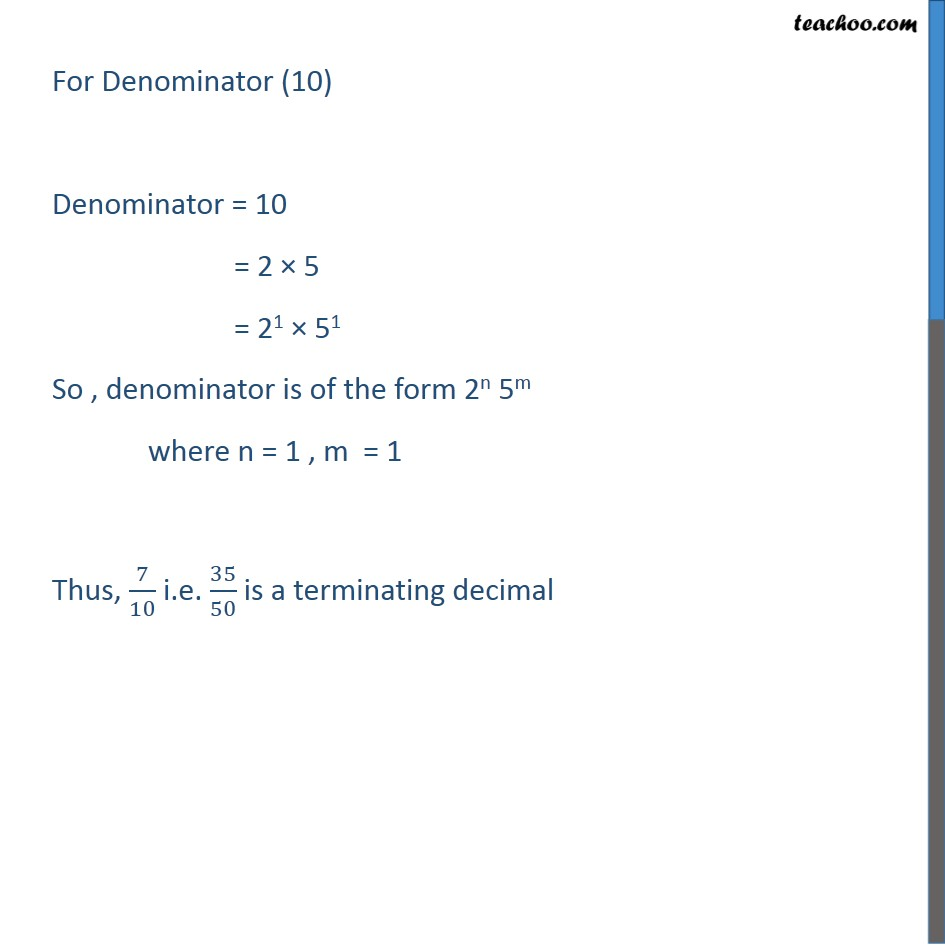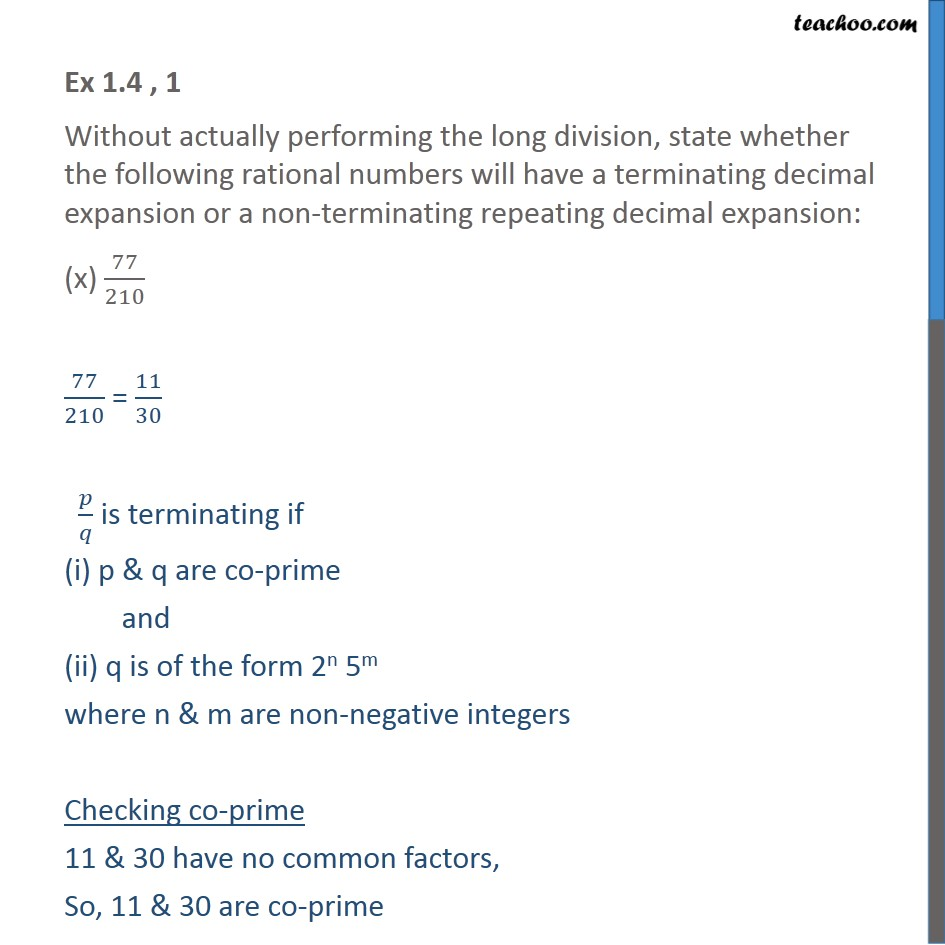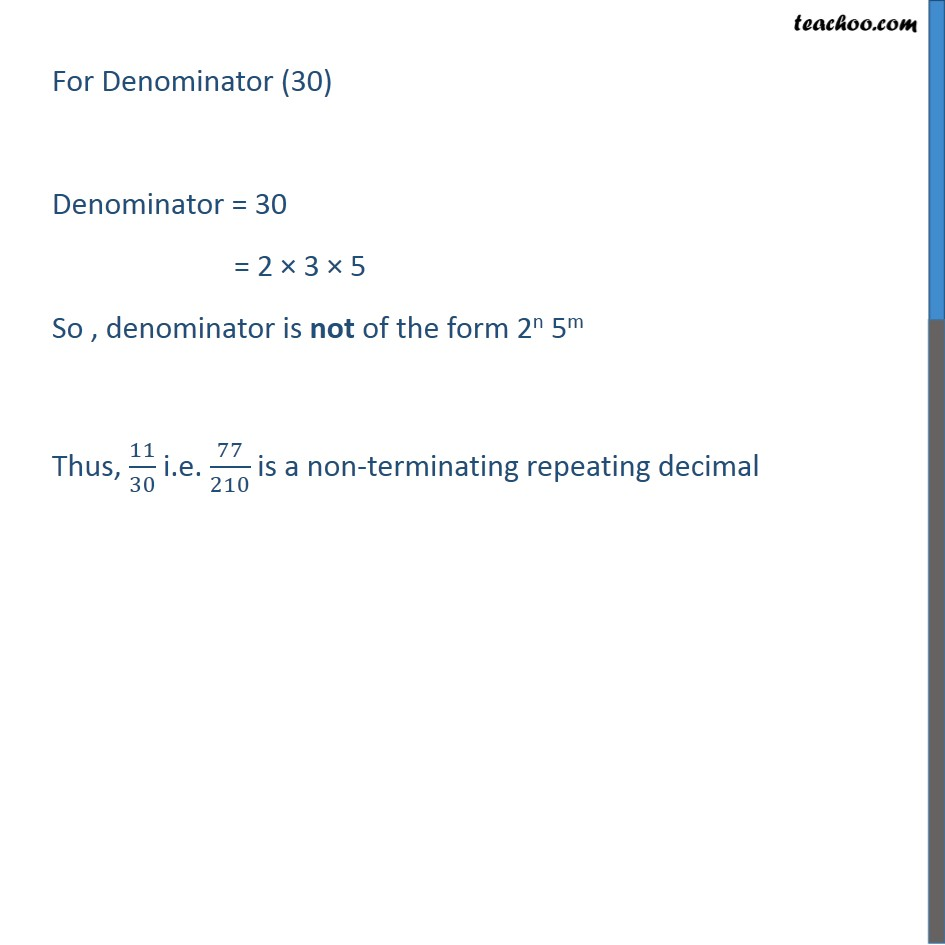1. Chapter 1 Class 10 Real Numbers
2. Concept wise
3. Decimal Expansion

Transcript

Ex 1.4 , 1 Without actually performing the long division, state whether the following rational numbers will have a terminating decimal expansion or a non-terminating repeating decimal expansion: 13/3125 𝑝/𝑞 is terminating if (i) p & q are co-prime and (ii) q is of the form 2n 5m where n & m are non-negative integers Checking co-prime 13 & 3125 have no common factors, So, 13 & 3125 are co-prime For Denominator (3125) Denominator = 55 = 1 × 55 = 20 × 55 So , denominator is of the form 2n 5m where n = 0 , m = 5 Thus , 13/3125 is a terminating decimal Ex 1.4 , 1 Without actually performing the long division, state whether the following rational numbers will have a terminating decimal expansion or a non-terminating repeating decimal expansion: (ii) 17/8 𝑝/𝑞 is terminating if (i) p & q are co-prime and (ii) q is of the form 2n 5m where n & m are non-negative integers Checking co-prime 17 & 8 have no common factors, So, 17 & 8 are co-prime For Denominator (8) Denominator = 23 = 1 × 23 = 50 × 23 So , denominator is of the form 2n 5m where n = 3 , m = 0 Thus, 17/8 is a terminating decimal Ex 1.4 , 1 Without actually performing the long division, state whether the following rational numbers will have a terminating decimal expansion or a non-terminating repeating decimal expansion: (iii) 64/455 𝑝/𝑞 is terminating if (i) p & q are co-prime and (ii) q is of the form 2n 5m where n & m are non-negative integers Checking co-prime 64 & 455 have no common factors, So, 64 & 455 are co-prime For Denominator (455) Denominator = 5 × 7 × 13 So , denominator is not of the form 2n 5m Thus, 64/455 has a non-terminating repeating decimal expansion Ex 1.4 , 1 Without actually performing the long division, state whether the following rational numbers will have a terminating decimal expansion or a non-terminating repeating decimal expansion: (iv) 15/1600 15/1600 = 3/320 𝑝/𝑞 is terminating if (i) p & q are co-prime and (ii) q is of the form 2n 5m where n & m are non-negative integers Checking co-prime 3 & 320 have no common factors, So, 3 & 320 are co-prime For Denominator (320) Denominator = 26 × 51 So , denominator is of the form 2n 5m where n = 6 , m = 1 Thus, 3/320 i.e. 15/1600 is a terminating decimal Ex 1.4 , 1 Without actually performing the long division, state whether the following rational numbers will have a terminating decimal expansion or a non-terminating repeating decimal expansion: (v) 29/343 𝑝/𝑞 is terminating if (i) p & q are co-prime and (ii) q is of the form 2n 5m where n & m are non-negative integers Checking co-prime 29 & 343 have no common factors, So, 29 & 343 are co-prime For Denominator (343) Denominator = 7 × 7 × 7 So , denominator is not of the form 2n 5m Thus, 29/343 has a non-terminating repeating decimal expansion Ex 1.4 , 1 Without actually performing the long division, state whether the following rational numbers will have a terminating decimal expansion or a non-terminating repeating decimal expansion: (vi) 23/(23 52) 𝑝/𝑞 is terminating if (i) p & q are co-prime and (ii) q is of the form 2n 5m where n & m are non-negative integers Checking co-prime 23 & 23 52 have no common factors, So, 23 & 23 52 are co-prime For Denominator (23 52 ) Denominator = 23 × 52 So , denominator is of the form 2n 5m where n = 3 , m = 2 Thus , 23/(23 52) is a terminating decimal Ex 1.4 , 1 Without actually performing the long division, state whether the following rational numbers will have a terminating decimal expansion or a non-terminating repeating decimal expansion: (vii) 129/(22 57 75) 𝑝/𝑞 is terminating if (i) p & q are co-prime and (ii) q is of the form 2n 5m where n & m are non-negative integers Checking co-prime 129 & 22 57 75 have no common factors, So, 129 & 22 57 75 are co-prime For Denominator (22 57 75) Denominator = 22 57 75 So , denominator is not of the form 2n 5m Thus, 129/(22 57 75) has a non-terminating repeating decimal expansion. Ex 1.4 , 1 Without actually performing the long division, state whether the following rational numbers will have a terminating decimal expansion or a non-terminating repeating decimal expansion: (viii) 6/15 6/15 = 2/5 𝑝/𝑞 is terminating if (i) p & q are co-prime and (ii) q is of the form 2n 5m where n & m are non-negative integers Checking co-prime 2 & 5 have no common factors, So, 2 & 5 are co-prime For Denominator (5) Denominator = 5 = 51 = 1 × 51 = 20 × 51 So , denominator is of the form 2n 5m where n = 0 , m = 1 Thus , 2/5 i.e. 6/15 is a terminating decimal Ex 1.4 , 1 Without actually performing the long division, state whether the following rational numbers will have a terminating decimal expansion or a non-terminating repeating decimal expansion: (ix) 35/50 35/50 = 7/10 𝑝/𝑞 is terminating if (i) p & q are co-prime and (ii) q is of the form 2n 5m where n & m are non-negative integers Checking co-prime 7 & 10 have no common factors, So, 7 & 10 are co-prime For Denominator (10) Denominator = 10 = 2 × 5 = 21 × 51 So , denominator is of the form 2n 5m where n = 1 , m = 1 Thus, 7/10 i.e. 35/50 is a terminating decimal Ex 1.4 , 1 Without actually performing the long division, state whether the following rational numbers will have a terminating decimal expansion or a non-terminating repeating decimal expansion: (x) 77/210 77/210 = 11/30 𝑝/𝑞 is terminating if (i) p & q are co-prime and (ii) q is of the form 2n 5m where n & m are non-negative integers Checking co-prime 11 & 30 have no common factors, So, 11 & 30 are co-prime For Denominator (30) Denominator = 30 = 2 × 3 × 5 So , denominator is not of the form 2n 5m Thus, 11/30 i.e. 77/210 is a non-terminating repeating decimal

Decimal Expansion